# NUC980 运行 RT-Thread 时使用 UART

## 板子上的 UART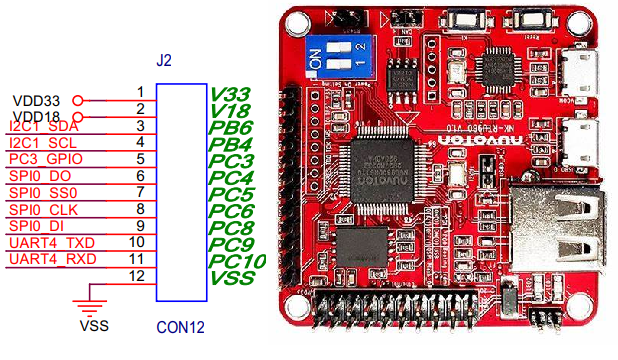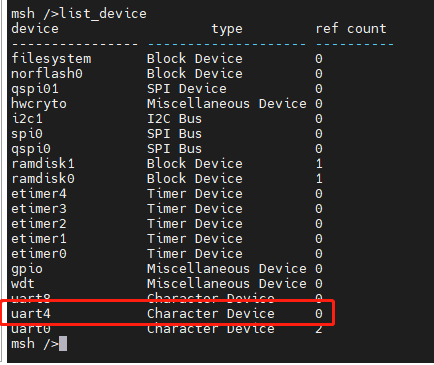## RT-Thread 中关于 uart 的 API

rt_device_find() 查找设备
rt_device_open() 打开设备
rt_device_write() 写入数据
rt_device_control() 控制设备
rt_device_set_rx_indicate() 设置接收回调函数
rt_device_set_tx_complete() 设置发送完成回调函数
rt_device_close() 关闭设备

## 发送

#include <drv_uart.h>

#define USER_UART_DEVNAME "uart4"

int uart4_test(int argc, char **argv)
{
rt_device_t serial;
char txbuf;
rt_err_t ret;
int str_len;

serial = rt_device_find(USER_UART_DEVNAME);
if (!serial)
{
rt_kprintf("Can't find %s. EXIT.\n", USER_UART_DEVNAME);
goto exit_test_uart4;
}

/* Interrupt RX */
ret = rt_device_open(serial, RT_DEVICE_FLAG_INT_RX);
RT_ASSERT(ret == RT_EOK);
rt_kprintf("Open uart success\r\n");
/* Say Hello */
ret = rt_device_write(serial, 0, "Hello NUC980 using uart4", rt_strlen("Hello NUC980 using uart4"));
ret = rt_device_close(serial);
RT_ASSERT(ret == RT_EOK);

exit_test_uart4:

return 0;
}
MSH_CMD_EXPORT(uart4_test, test uart4 write);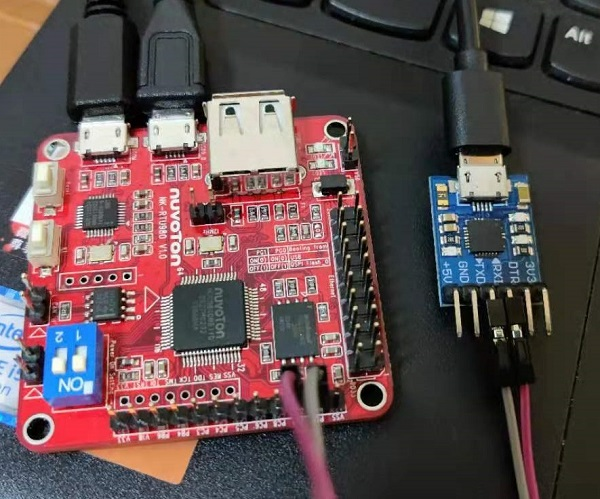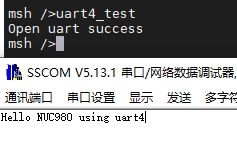## 接收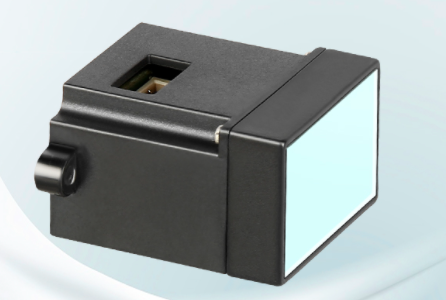TOF 跟 NuMaker-RTU-NUC980 连接为：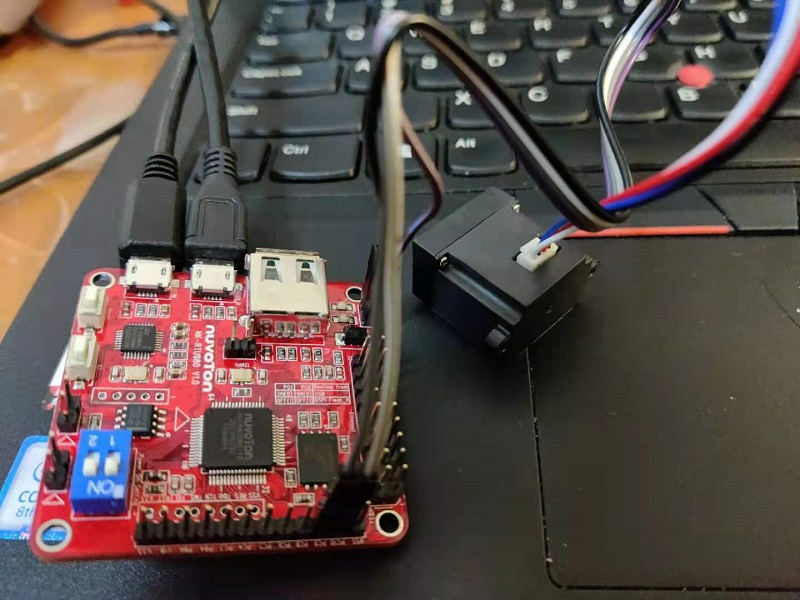#include <rtthread.h>

#define SAMPLE_UART_NAME       "uart4"      /* 串口设备名称 */

/* 用于接收消息的信号量 */
static struct rt_semaphore rx_sem;
static rt_device_t serial;

/* 接收数据回调函数 */
static rt_err_t uart_input(rt_device_t dev, rt_size_t size)
{
/* 串口接收到数据后产生中断，调用此回调函数，然后发送接收信号量 */
rt_sem_release(&rx_sem);

return RT_EOK;
}

static int read_tof_skz(int argc, char *argv[])
{
rt_err_t ret = RT_EOK;
uint8_t rx_buf;
uint8_t rx_index = 0;
uint16_t count =0 ;
uint8_t i=0;

uint8_t temp = 0;
uint16_t value = 0;
char ch;
/* 查找串口设备 */
serial = rt_device_find(SAMPLE_UART_NAME);
if (!serial)
{
rt_kprintf("find %s failed!\n", SAMPLE_UART_NAME);
return RT_ERROR;
}

/* 以中断接收及轮询发送方式打开串口设备 */
rt_device_open(serial, RT_DEVICE_FLAG_INT_RX);
/* 初始化信号量 */
rt_sem_init(&rx_sem, "rx_sem", 0, RT_IPC_FLAG_FIFO);
/* 设置接收回调函数 */
rt_device_set_rx_indicate(serial, uart_input);
while (1)
{
/* 从串口读取一个字节的数据，没有读取到则等待接收信号量 */
while (rt_device_read(serial, 0, &ch, 1) != 1)
{
/* 阻塞等待接收信号量，等到信号量后再次读取数据 */
rt_sem_take(&rx_sem, RT_WAITING_FOREVER);
}
rt_device_write(serial, 0, &ch, 1);
if( ch == '~')
{
rx_index = 0;
}

rx_buf[rx_index ] = ch;
if(rx_index >=1)
{
if(rx_buf[rx_index] == '\n' && rx_buf[rx_index-1] == '\r' )
{
// 9
value = 0;
temp = rx_buf[rx_index - 9] - '0';
value = (value + temp <<24);

temp = rx_buf[rx_index - 8] - '0';
value = (value + temp <<16);

temp = rx_buf[rx_index - 7] - '0';
value = (value + temp <<8);

temp = rx_buf[rx_index - 6] - '0';
value = (value + temp );
rt_kprintf("Value is:%d\r\n",value);

count ++;
}
}
rx_index ++;
if(rx_index >=19)
rx_index = 0;

if(count > 2500)
break;
}
rt_device_close(serial);
return ret;
}
/* 导出到 msh 命令列表中 */


/* 设置接收回调函数 */
rt_device_set_rx_indicate(serial, uart_input);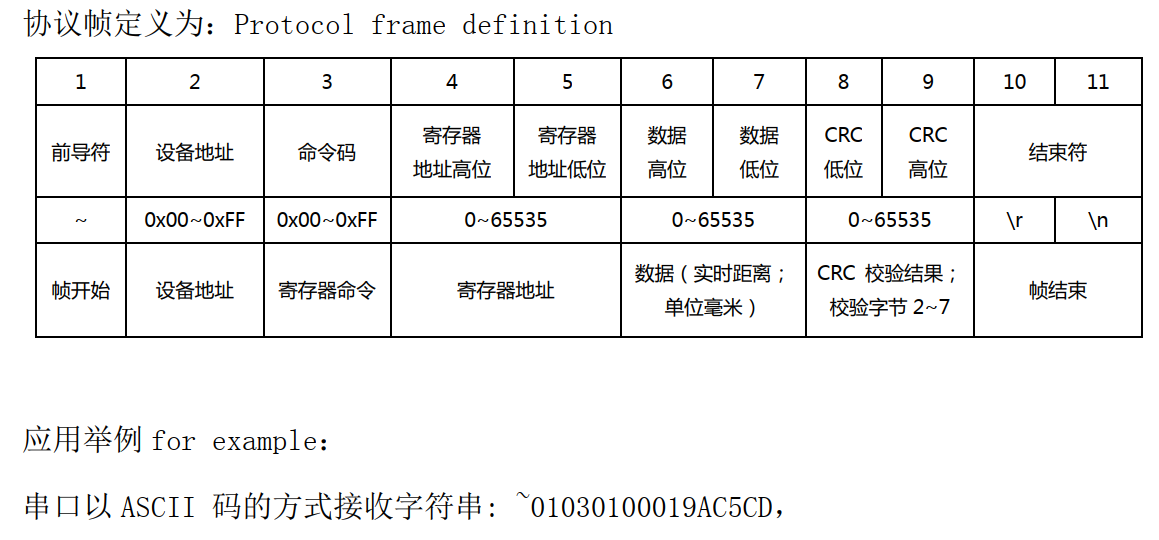if(rx_buf[rx_index] == '\n' && rx_buf[rx_index-1] == '\r' )
{
// 9
value = 0;
temp = rx_buf[rx_index - 9] - '0';
value = (value + temp <<24);

temp = rx_buf[rx_index - 8] - '0';
value = (value + temp <<16);

temp = rx_buf[rx_index - 7] - '0';
value = (value + temp <<8);

temp = rx_buf[rx_index - 6] - '0';
value = (value + temp );
rt_kprintf("Value is:%d\r\n",value);

count ++;
}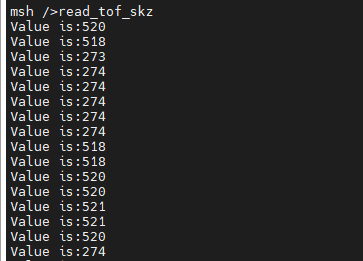posted @ 2021-07-01 15:30  哈拎  阅读(623)  评论(0编辑  收藏  举报